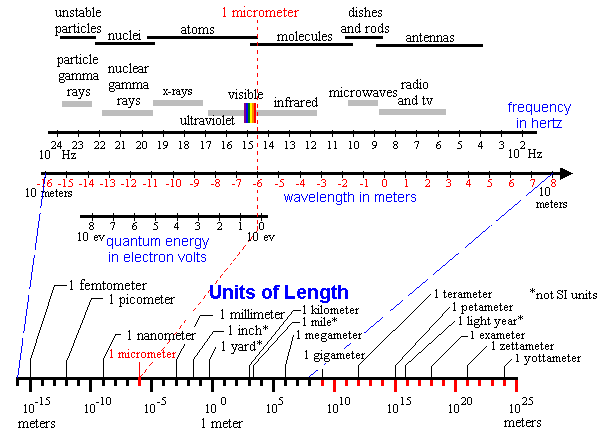## Logarithmic Scales

The chart below is designed to show the basic physical relationship between energy and electromagnetic radiation. A fundamental result of this relationship is that the smaller an object, the smaller is the wavelength of radiation required to form an image of it. Furthermore, the smaller the wavelength, the greater the energy required to produce it. This is why larger and more powerful accelerators are required to discover smaller and more energetic particles.

The top row of bars indicates the source for electromagnetic radiation of a particular frequency range. The second row of bars gives the name associated with a specific range of frequencies. The next three lines are the three related scales of frequency, wavelength, and energy. The bottom scale provides the names of some commonly used units along the distance scale.

Electromagnetic radiation with a wavelength of one micrometer, lies just redward (longer wavelength) of what the eye can see. It has a frequency of 3.00 x 1014 hertz and a quantum energy of 1.24 electron volts. This single example will fix the relation between the three scales, for frequency is strictly proportional to quantum energy and inversely proportional to wavelength. (The wavelength scale--and other red markers--can be used to jump to the appropriate power of ten.)